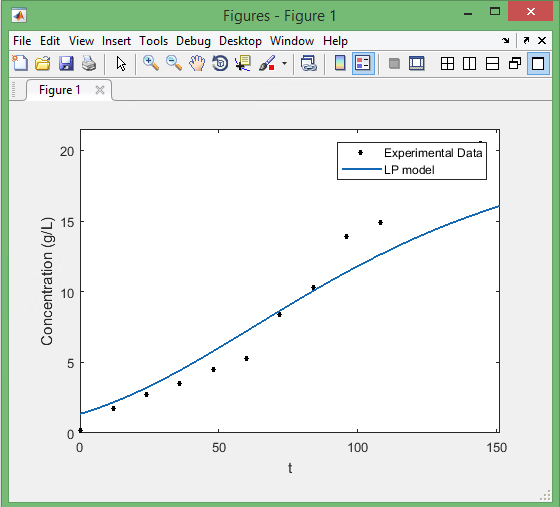How to extract figure data in MATLABRecently, I had to deal with a set of data, some from experiments, and some obtained using MATLAB “Curve Fitting Tool”. As much as I like MATLAB figures, I usually find Excel features more desirable for good-looking plots! So, I needed to extract the data of Curve Fitting Tool. Here is what I did:

Save the figure as a “.fig” file.

Make a m-file with the following code to get the x, y data of the curve!

Then you have access to the data, and you can get the arrays as